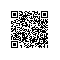# Consecutive sequence with sum 0

You are given an integer array which contains positive integers, zero and negetive integers
count how many consecutive sequences in this array make a sum of 0.

exmaple
int[] a = {4, -1, 2, 1, -2, -1, 5, 0} ;
The result is 2
-1, 2, 1, -2, makes a sum of 0
2, 1, -2, -1, makes a sum of 0
0 makes a sum of 0

consider a consecutive sequence in array a, say xi, ... xj, if xi + ... + xj = 0, then x0 + .. + x(i-1) = x0 + .. + xj
so we can compute all the prefix sum of array a, and see whether there are equal elements among these sum
if there is, then there must be some consecutive sequence, makes sum of 0Code使用钉钉扫一扫加入圈子
+ 订阅